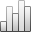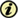Acta Stereologica

0351-580XMore Statisticssince 05 December 2013 :
View(s): 266 (3 ULiège)
Manuel Amaral Fortes

### A general method to solve the integral equations of stereology

(Volume 10 (1991) — Number 2 - Stermat '90 (part II) - Dec. 1991)
Article#### Abstract

The scope of stereology is to infer characteristics of features of a three-dimensional structure from measurements on two-dimensional (usually planar) sections. A feature is characterized by one or more variables, x, with a probability distribution function X(x). In a two-dimensional section one observes "prints" of the three-dimensional features intersected, which are characterized by variables, y, with a measurable distribution Y(y). Provided that the variables, x, completely define the features, it is possible to derive a relation between the two distributions, in the form of an integral equation giving explicity Y(y) as an integral containing X(x). The integral equation is usually unsolvable for X(x) by analytical means.

In the paper, a general method is developed to solve equations of this type. The basis of the method is the discretization of the two distributions in classes, or intervals, and the linearization of the relation between them, which can then be solved for X(x) by standard matrix methods. The linear relation between the two distributions involves coefficients which can be calculated with any degree of accuracy but depend on the chosen amplitudes of the intervals.

The stability of the solutions can easily be assessed through the method developed, and various parameters are introduced with which the stability can be evaluated.

The method is applied to the determination of the length distribution of oriented lines in a plane from the measurement of the number of points of intersection with test lines of various orientations.

Keywords : integral equations, stability of solutions, stereology